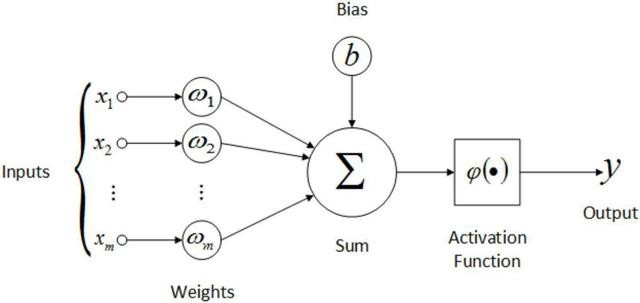# What is an Activation Function and its types?

Activation Function

The activation function is a node that is put at the end of or in between Neural Networks . They help to decide if the neuron would fire or not. “The activation function is the non linear transformation that we do over the input signal. This transformed output is then sent to the next layer of neurons as input.”
ref: https://medium.com/dataseries/basic-overview-of-convolutional-neural-network-cnn-4fcc7dbb4f17

Well an activation function is the mathematical function which is used in the last node of a neural network which is for prediction.

Below the image show a basic neural network architecture.There are many types of activation functions but the one which are mostly used are:

1. Sigmoid function
2. ReLU function
3. tanh function
4. Leaky ReLU